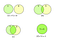Linear Regression deals with modeling a linear relationship between a dependent variable and several explanatory variables. Essentially, we want to fit a line through our data points in space.

But there can be multiple possible lines. Thus to find the best line, there is a need for a measure of goodness of fit, ergo the Coefficient of Determination — R².

Before you proceed, here is a list of 5 must-read articles on Regression to brush up your basics:

You can also check out (and participate in) a detailed discussion on this question with answers from other data science experts here:

Coefficient of Determination — R²

Consider the case of simple regression as shown in the figure below.As explained in Gujarati, Damodar N. Basic econometrics. Tata McGraw-Hill Education, 2009.

Circle Y represents the variation in our dependent variable, circle X, in our independent variable.

The overlap of the circles represents the extent to which the variation in Y is explained by the variation in X.

The coefficient is the numerical measure of this overlap and is called the Coefficient of Determination. This becomes the Multiple Coefficient of Determination in the case of Multiple Regression.

To state formally,

R² measures the proportion of the total variation in Y explained by the regression model.

This variation is measured in terms of the sum of squared errors from the mean.

If we have n observations (X_i, Y_i) and f(.) is our predictor function,

Here are some key points about R²:

• It is a non-negative quantity with range 0 ≤ R² ≤ 1
• R² = 0 implies that the regression line does not fit the data at all.
• R² = 1 implies that the regression line is a perfect fit.

Problem with R² — Value increases with the number of explanatory variables

Think about it. R² is the ratio of the explained variance to the total variance. On adding a new variable the explained variance and hence the value of R² will increase, or at least, will not decrease.

However, this does not at all mean that the model with the added variable is better than the model without it. R² can be misleading if used to compare models with a different number of predictors.

Adjusted R² is a modified version of R² adjusted with the number of predictors. It penalizes for adding unnecessary features and allows a comparison of regression models with a different number of predictors.

Here k is the number of explanatory variables in the model and n is the number of observations.

The value of adjusted R² is always less than that of R².

The adjusted R-squared increases only if the new term improves the model more than would be expected by chance. It decreases when a predictor improves the model by less than expected by chance.

Also, note that the value of adjusted R² can be negative.

Obtaining a negative value for Adjusted R² can indicate few or all of the following:

• The linear model is a poor fit for the data
• The number of predictors is large
• The number of samples is small

R² and Adjusted R² in Python

Generate a random dataset first

X has 6 features. This is how the dependent variable y varies with each of these features:

y versus X or y versus X could possibly be linear relationships. Let us fit a line using Ordinary Least Squares Regression between y and X using the statsmodels library in Python. The summary() function can be used to view the R² and Adjusted R² coefficients.

Here is the summary:

Now add another explanatory variable, X, to see the effect on both the coefficients.

Interesting! The value of R² increased while the value of adjusted R² dropped. X is an insignificant feature to add to the linear relationship. This is further confirmed on finding the adjusted R² for the OLS regression on Y versus X individually. We observe that it is negative.

You can also use the r2_score function from the metrics module in sklearn. However, there is no such function to find the adjusted r2_score. We need to use its formula to calculate it.

Here is the regression analysis of the famous mtcars dataset without the categorical variables in sklearn:

We obtain an R² score of about 0.81 and an adjusted R² score of about 0.77

End Notes

Although both R² and adjusted R² are measures of goodness of fit, linear regression models should not be built with the sole goal of maximizing these coefficients. This will eventually tempt us to introduce many insignificant predictors in our model. As a result, our model will be inaccurate and unreliable.

Written by

Khyati Mahendru

Analytics Vidhya is a community of Analytics and Data Science professionals. We are building the next-gen data science ecosystem https://www.analyticsvidhya.com

Welcome to a place where words matter. On Medium, smart voices and original ideas take center stage - with no ads in sight. Watch
Follow all the topics you care about, and we’ll deliver the best stories for you to your homepage and inbox. Explore
Get unlimited access to the best stories on Medium — and support writers while you’re at it. Just \$5/month. Upgrade